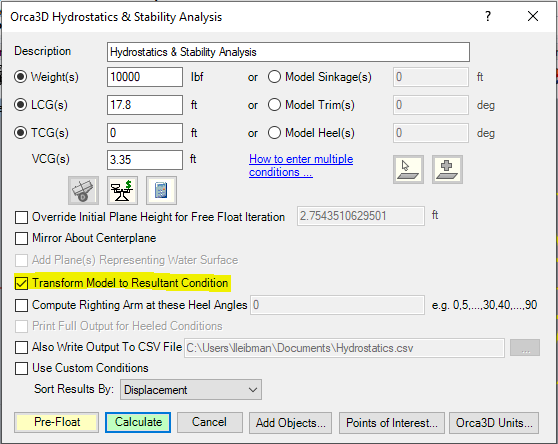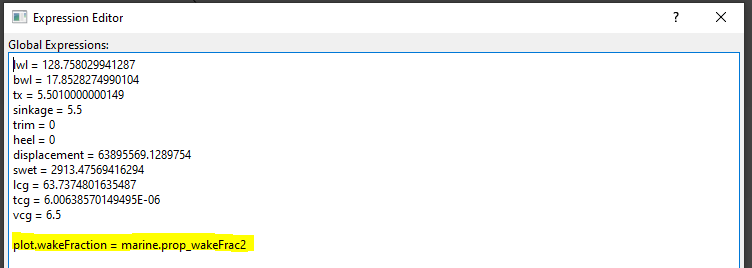Detailed propeller design often relies on a description of the inflow wake distribution in the plane of the propeller in order to properly design the blade shapes to maximize efficiency, minimize cavitation, and reduce the effects of the unsteady propeller forces (like noise and vibrations). For many years scale model testing has been used to predict the wake distribution by running tests either in a towing tank or a flow channel over a range of vessel speeds and using a measurement device (such as pitot tubes or Laser Doppler Velocimetry ) to measure the local flow velocities in the plane of the propeller. It is possible to use Orca3D Marine CFD to simulate and measure the wake distribution. This approach has the benefit of not being limited to Reynolds numbers associated with smaller scale models since the CFD simulations can be run at full scale. The simulation setup can be performed using either a fully manual approach or a semi-automated approach as described below.

Manual Approach

To simulate a propeller wake survey with Orca3D Marine CFD using the manual approach, the following steps should be performed:

1. First generate one or more CFD resistance simulations at the speeds of interest in the way you normally would with Orca3D Marine CFD. Wake measurements are traditionally made in resistance simulations with appendages but in the absence of the propeller.

2. The wake survey process relies on distributing a series of "monitoring points" in SimericsMP over the plane of the propeller. As the name implies monitoring points allow the user to monitor the local flow behavior, including flow velocities, at specific locations. Because the determination of monitoring point locations is more easily accomplished in a CAD tool like Rhino, we start by opening the Rhino model containing the geometry used to generated the CFD simulation. Start by first transforming the Rhino model so that the z = 0 plane is the static flotation plane. This is done to match the geometry transformation that is applied in the CFD simulation. To do this, run the OrcaHydrostatics command, enter the mass properties or flotation plane at which the simulations are being performed and check the box that says "Transform Model to Resultant Condition" as shown below.3. Next, create points distributed over the propeller plane as shown below. Here Rhino was used to draw a line from bottom to top of the propeller disk (note this line is not vertical but raked and perpendicular to the shaft line) as well as a line from port to starboard sides of the propeller disk. Then points were evenly distributed along these lines representing here increments of 20% of the propeller diameter. Of course you can use more or less point as desired as well as additional line segments as needed.4. With Rhino still open, also open the CFD simulation in SimericsMP. As shown below use the highlighted toolbar button in the Geometric Entities panel to create as many monitoring points as you have points in the Rhino model. For clarity, in the figure below we only created the 11 monitoring points ("Point -5" through "Point 5") distributed along the line segment from bottom to top of the propeller disk.

a. Select one of the monitoring points in the Geometric Entities panel and then in the Geometry tab of the Properties panel change the Type to Prescribe Motion. We will specify the point location so that it moves with the heave and pitch of the vessel. Measure the corresponding point location in Rhino using the EvaluatePt command. This will provide the x, y, z coordinates of the point in the same coordinate system as SimericsMP since you transformed the model. However, this will be prior to any dynamic pitch and heave. Open the Expressions Editor and define an expression for the location of the point. The expression takes the measured position of the point in static equilibrium from Rhino, then rotates by the pitch angle about the CG then raises the point by the heave magnitude. You'll notice that for some of the points we multiplied the Rhino location by 0.3048 because the Rhino model is in ft units and the Simerics model is in SI units.

b. Close the expression editor and set the x, y, z values of the selected monitoring point to the associated expression variable. So for Monitoring Point "Point 0" we set the coordinates to [pt0.x, pt0.y, pt0.z] corresponding to the expression for that point. Now the point will move with the vessel.5. Start the simulation. While the simulation is running create a new X-Y Plot. Select the points in the Geometric Entities panel, click Velocity X in the plot variables and click the Plot Selected Variables button to add these to the plot as shown below. The plotted values represent the flow velocity associated with each monitoring point. Note that the velocity is relative to the free stream velocity. So in the plot below, points -2 through -5, which are below the propeller centerline, are all clustered around 0 kt indicating that the wake fraction there is close to 1. On the other side points 2 through 5, which are above prop centerline are around -9 to -10 kt relative to the free stream velocity of 25 kt, yielding local wake fraction of around 15/25 or 0.6. This is because these point locations are right behind the shaft strut where there is a significant wake deficit. To get a nominal wake fraction you would average all of the points radially and circumferentially.Finally the image below shows the dynamic pressure on the model along with monitoring points -5, 0, and 5 circled in black. The computed values of pressure and velocity components are output to the file *_points.txt in the Simerics project folder. You can use Excel to import and process these results.Semi-Automated Approach

To simulate a propeller wake survey with Orca3D Marine CFD using the semi-automated approach, the following steps should be performed:

1. As in the manual approach, first generate one or more CFD resistance simulations at the speeds of interest in the way you normally would with Orca3D Marine CFD. Wake measurements are traditionally made in resistance simulations with appendages but in the absence of the propeller.

2. As before, this approach uses monitoring points, but in this case the locations of the monitoring points are automatically computed for you. Once you open the resistance simulation in SimericsMP there is an option to set up a wake survey by adding a special Propulsion Source. As shown in the image below first select the "Marine" module in the Model panel and then in the Properties panel expand the Propulsion Option. Click on the dropdown and choose "Add Wake Survey" to add a new Wake Survey. This type of propulsion source is a special type in that it does not add any propulsive force to the simulation but instead allows some of the same inputs that are used to define a propeller to be specified along with others to define the disk within which the wake survey is desired.Once you've added the wake survey source, expanding it reveals the required input parameters as shown below. This consists of an integer ID, the "Position" which is generally the center of the propeller disk, the "Direction" defining the vector thrust direction of the propeller (x = -1 is forward), and the "Wake" and "Hub" diameters where the former is generally the same as the propeller diameter. Remember the the position and direction inputs are in the initial condition which corresponds to the static equilibrium condition computed in Orca. You can use the same technique of transforming the model to the resultant flotation condition described above in the "Manual Approach" if you would like to measure the location directly in Rhino. Two additional inputs include the number of monitoring points to create within the disk both radially and circumferentially.After that is done you can select the "Preview Monitor Points" dropdown and select Yes to create the points. Alternatively they will be automatically created when you run the simulation.

This is all that is required to define the locations of the monitoring points. However, one additional feature of the semi-automated approach described here is that SimericsMP will automatically perform an integration of the longitudinal flow velocities at each point to determine an average or "nominal" wake fraction. To plot the computed wake fraction you will need to define a custom plot variable as shown below. Note that the integer value "2" in that expression must match the ID of the WakeSurvey. This allows for multiple wake survey sources to be added if needed.